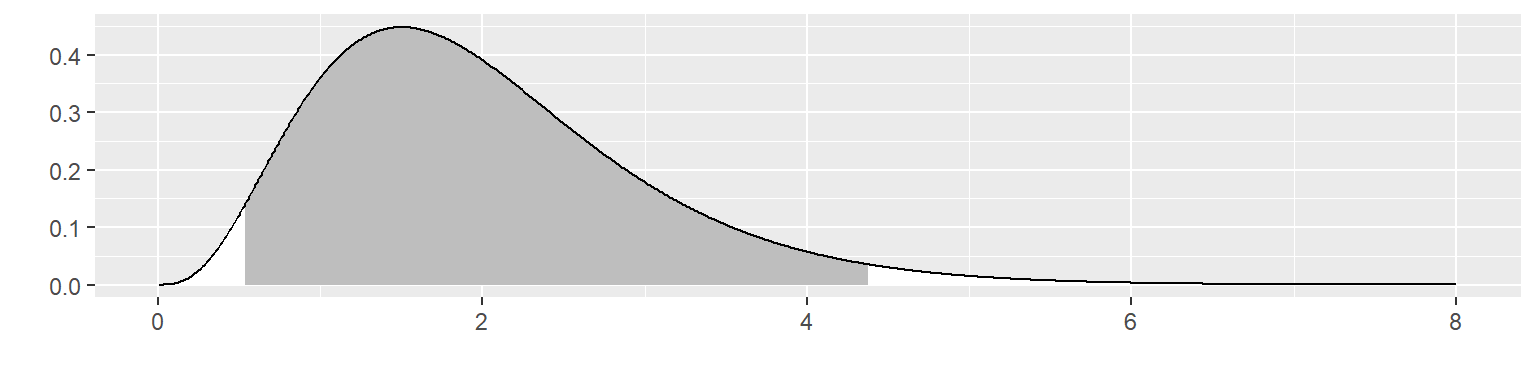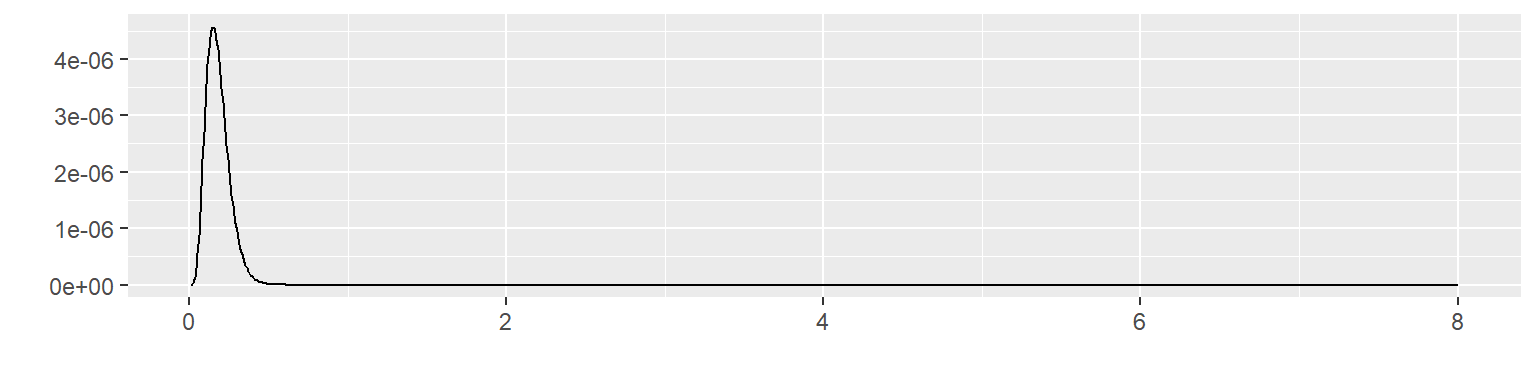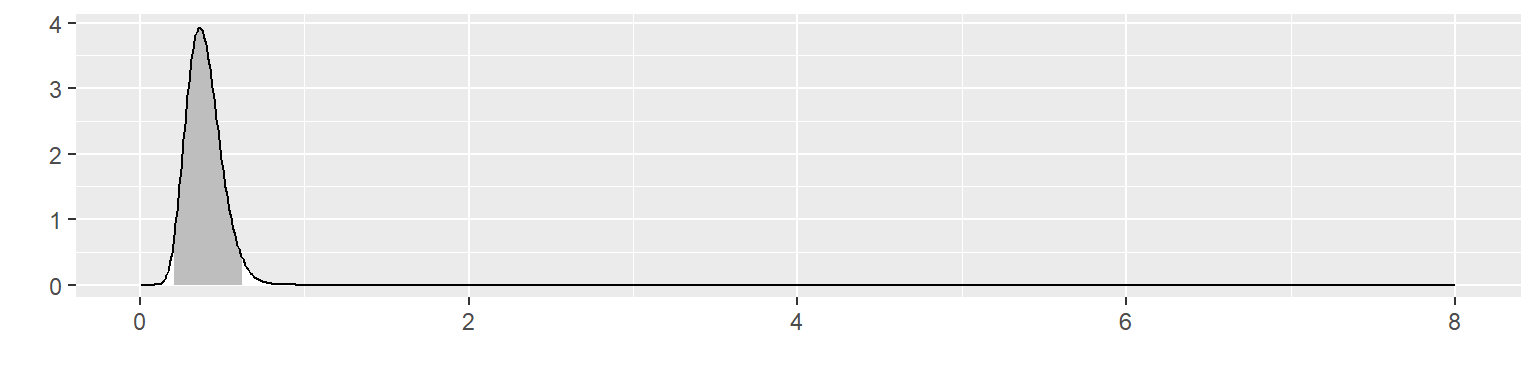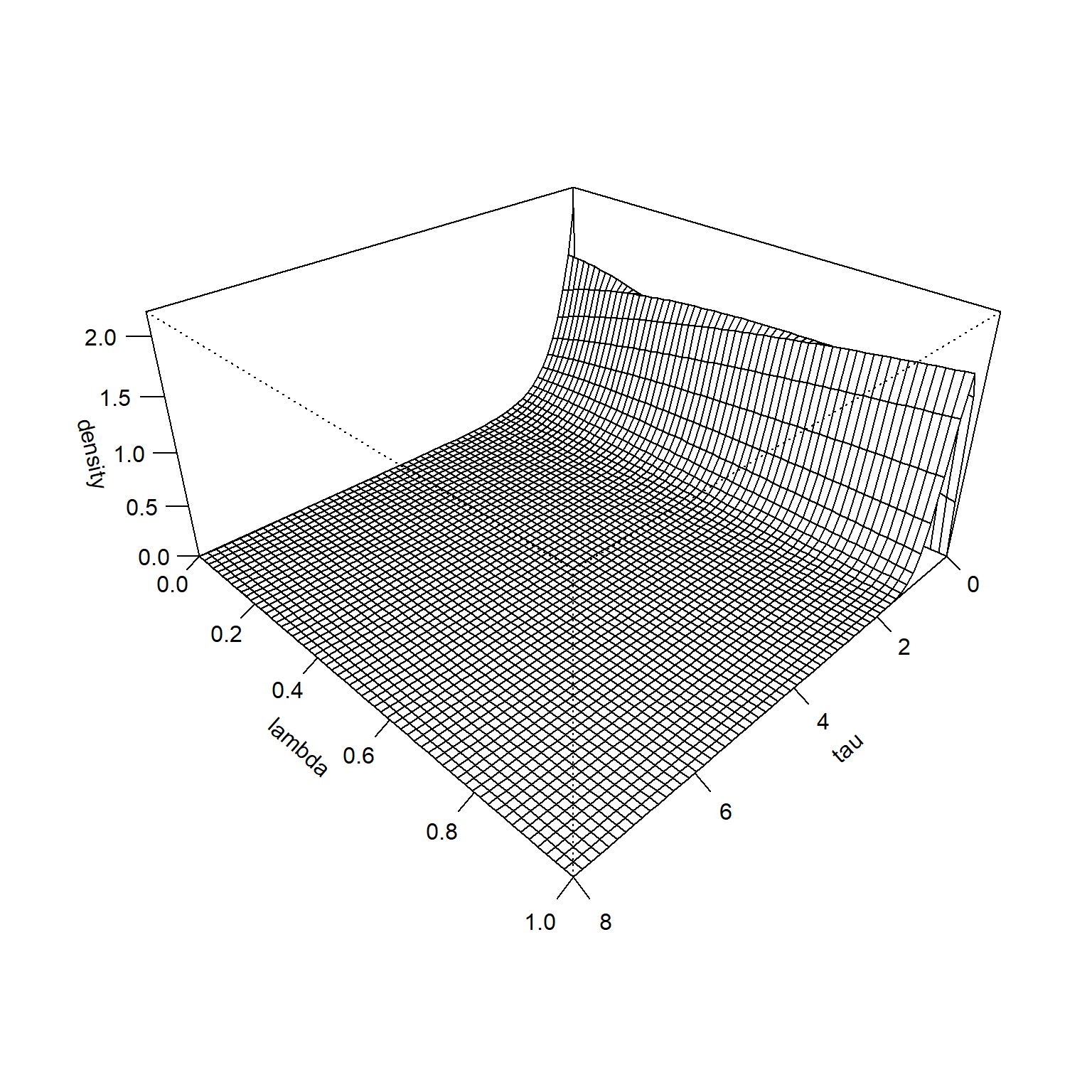# The hedging hyperprior for the normal distribution: known mean, unknown precision

## 2023-03-14

suppressMessages(suppressWarnings(library(MASS)))
suppressMessages(suppressWarnings(library(tidyverse)))
knitr::opts_chunk$set(echo=TRUE, fig.width=8, fig.height=2) I want to write a paper about the hedging hyperprior. I need to work out some simple examples first. Here is an example involving the normal distribution. ### A simple Bayesian analysis with an informative prior. There are lots of guides on the Internet on using a normal prior for data that is normally distributed with the population standard deviation $$\sigma$$ known or unknown. I used papers written by Kevin P. Murphy and Michael I. Jordan to get these formulas. For simplicity, all sums and products are assumed to go from i=1 to n. Consider a Bayesian analysis of an outcome variable that has a normal distribution with known mean and unknown precision. Assume that both the mean and the precision of the normal distribution are unknown. Other cases with mean unknown and precision known and with both mean and precision unknown appear on a different web pages. Assume that the mean of the normal distribution, $$\mu$$, is known and equal to $$\mu_F$$. An earlier case with precision known and the mean unknown appears on a different web page. A more general case, with both the mean and the precision of the normal distribution unknown is also described on a separate web page. For simplicity, we will drop any multiplicative constants not involving X or $$\tau$$ $$f(X; \tau) \propto \sqrt{\tau} e^{-\frac{1}{2}\tau(X-\mu_F)^2}$$ The conjugate prior for a normal distribution is a gamma distribution. $$g(\tau; \alpha_0, \beta_0) = \frac {\beta_0^{\alpha_0}} {\Gamma(\alpha_0)} \tau^{\alpha_0-1} e^{-\beta_0 \tau}$$ Set the prior parameters to $$\alpha_0=n_0$$ $$\beta_0=n_0\tau_0$$ and remove any multiplicative constants with respect to $$\tau$$ to get $$g(\tau; n_0, \tau_0) \propto \tau^{n_0-1} e^{-n_0 \tau_0 \tau}$$ In this parameterization, $$n_0$$ is the prior sample size. You have collected a sample of n data points, $$\mathbf{X} = X_1, X_2, ..., X_n$$. This gives you a likelihood of $$L(\mathbf{X} | \mu) \propto \tau^{n/2} e^{- \frac{1}{2} \tau\sum(X_i-\mu_0)^2}$$ The posterior distribution is proportional to the product of the prior and the likelihood. $$h(\tau | \mathbf{X}) \propto \tau^{n_0-1} e^{-n_0 \tau / \tau0} \tau^{n/2} e^{- \frac{1}{2} \tau\sum(X_i-\mu_0)^2}$$ which can be rewritten as $$h(\tau | \mathbf{X}) \propto \tau^{n/2 + n_0-1} e^{-(n_0 \tau0 + \frac{1}{2}\sum(X_i-\mu_0)^2)}$$ which is a gamma distribution with posterior parameters $$\alpha_1 = n/2+n_0$$ and $$\beta_1 = n_0 \tau_0 + \frac{1}{2}\sum(X_i-\mu_0)^2)$$ ### A simple numerical example x_max <- 8 n0 <- 4 tau0 <- 0.5 alpha0 <- n0 beta0 <- n0*tau0 muF <- 2 n <- 10 x <- muF+ round(rnorm(n), 1)/tau0 ss <- sum((x-muF)^2) alpha1 <- alpha0 + n beta1 <- beta0 + 0.5*ss x_grid <- seq(0.01, x_max, length=1000) f <- function(x, mu, tau) { sqrt(tau/2*pi)*exp(-0.5*tau*(x-mu)^2) } g <- function(x, alpha, beta) { beta^alpha/gamma(alpha)*x^(alpha-1)*exp(-beta*x) } prior05 <- qgamma(0.025, alpha0, beta0) prior95 <- qgamma(0.975, alpha0, beta0) post05 <- qgamma(0.025, alpha1, beta1) post95 <- qgamma(0.975, alpha1, beta1)  Let’s illustrate this with an informative prior with $$n_0$$ = 4 and $$\tau_0$$ = 0.5. g_grid <- g(x_grid, alpha0, beta0) outside <- x_grid < prior05 | x_grid > prior95 x_color <- ifelse(outside, "white", "gray") data.frame(x=x_grid, y=g_grid) %>% ggplot(aes(x, y)) + geom_segment(aes(xend=x, yend=0), color=x_color) + geom_line() + xlab(" ") + ylab(" ")This corresponds to a fairly informative prior distribution. This distribution places $$\tau$$ within 0.54 and 4.38 with 95% probability. Let’s see what happens with this informative prior if you observe 10 values of x: -2, 0.2, -3, 2.2, 3.6, 1.2, 3.4, 3, 1, -1.6. The likelihood is f_grid <- rep(NA, length(x_grid)) for (i in 1:length(x_grid)) { f_grid[i] <- prod(f(x, muF, x_grid[i])) } x_color <- "white" data.frame(x=x_grid, y=f_grid) %>% ggplot(aes(x, y)) + geom_line() + xlab(" ") + ylab(" ")Multiply these two together to get the posterior distribution. h_grid <- g(x_grid, alpha1, beta1) outside <- x_grid < post05 | x_grid > post95 x_color <- ifelse(outside, "white", "gray") data.frame(x=x_grid, y=h_grid) %>% ggplot(aes(x, y)) + geom_segment(aes(xend=x_grid, yend=0), color=x_color) + geom_line() + xlab(" ") + ylab(" ")The posterior distribution is not close to either the prior distribution or the likelihood. It places $$\tau$$ within 0.22 and 0.65 with 95% probability. It excludes the prior mean of 0.5 and it excludes 7.1555556, the mean of the data. ### The hedging hyperprior When the likelihood of your data and your informative prior are in conflict, you may wish to downweight the informative prior. You can do this by including a hedging hyperprior. Define your prior distribution as a gamma distributions with prior mean $$\tau_0$$ and prior precision $$\alpha = 1 + \lambda(n0-1)$$$= _0 $where $$\lambda$$ ranges between 0 and 1. When $$\lambda=0$$, the prior precision reduces to $$alpha=1$$ which is equivalent to a very diffuse prior. When $$lambda=1$$, the prior precision is $$\alpha=\alpha_0$$ or the full strength prior. Place a hyperprior on the parameter $$\lambda$$. The uniform(0,1) distribution is a reasonable choice for the hyperprior, but others are possible. knitr::opts_chunk$set(echo=TRUE, fig.width=8, fig.height=8)
lambda_grid <- seq(0, 1, length=60)
tau_grid <- seq(0, x_max, length=60)

gstar <- function(tau, lambda) {g(tau, 1+lambda*(alpha0-1), (1+lambda*(alpha0-1))*beta0)}
density <- outer(tau_grid, lambda_grid, gstar)
op <- par(bg = "white")
z_max <- max(density, na.rm=TRUE)
persp(
tau_grid,
lambda_grid,
density,
zlim=c(0, 1.1*z_max),
xlab="tau",
ylab="lambda",
theta = 135,
phi = 30,
expand=0.5,
ticktype="detailed")This is the joint prior distribution of $$\tau$$ and $$\lambda$$.

It is hard to see at this scale, but the prior distribution is higher for small values of $$\lambda$$ when $$\tau$$ is very large or very small. This becomes more obvious if you remove the middle of the surface and rescale the Z-axis.

density <- outer(tau_grid, lambda_grid, gstar)
density[16:44, ] <- NA
op <- par(bg = "white")
z_max <- max(density, na.rm=TRUE)
persp(
mu_grid,
lambda_grid,
density,
zlim=c(0, 1.1*z_max),
xlab="tau",
ylab="lambda",
theta = 135,
phi = 30,
expand=0.5,
ticktype="detailed")

The likelihood function is constant across all values of $$\tau$$, so its surface looks like the following.

lambda_grid <- seq(0, 1, length=60)
tau_grid <- seq(0, x_max, length=60)

fstar <- function(mu, lambda) {f(xbar, mu, tau0)^n}
likelihood <- outer(mu_grid, lambda_grid, fstar)
z_max <- max(likelihood, na.rm=TRUE)
persp(
mu_grid,
lambda_grid,
likelihood,
zlim=c(0, 1.1*z_max),
xlab="mu",
ylab="lambda",
theta = 135,
phi = 30,
expand=0.5,
ticktype="detailed")

The product of the prior and the likelihood is proportional to the posterior distribution.

lambda_grid <- seq(0, 1, length=60)
mu_grid <- seq(0, x_max, length=60)

hstar <- function(mu, lambda) {g(mu, mu0, lambda*(tau0-tauF) + tauF)*f(xbar, mu, tauF)^n}
density <- outer(mu_grid, lambda_grid, hstar)
z_max <- max(density, na.rm=TRUE)
persp(
mu_grid,
lambda_grid,
density,
zlim=c(0, 1.1*z_max),
xlab="mu",
ylab="lambda",
theta = 135,
phi = 30,
expand=0.5,
ticktype="detailed")

A contour plot shows where the probability is concentrated. The labels on the contour lines represent how much probabilty is found inside the contour line.

density <- density / sum(density, na.rm=TRUE)
density %>%
as.vector %>%
sort %>%
rev -> sorted_density
cumulative_p <- 0
for (p in sorted_density) {
cumulative_p <- p + cumulative_p
density[density==p] <- cumulative_p
}
contour(
mu_grid,
lambda_grid,
density,
labcex=1,
levels=c(50, 75, 90)/100,
xlab="tau",
ylab="lambda")

You can see that the hedging hyperprior did its job. The most probable values of $$\lambda$$ are small and the prior mean (0.5) is largely ignored in favor of the mean of the data (??).